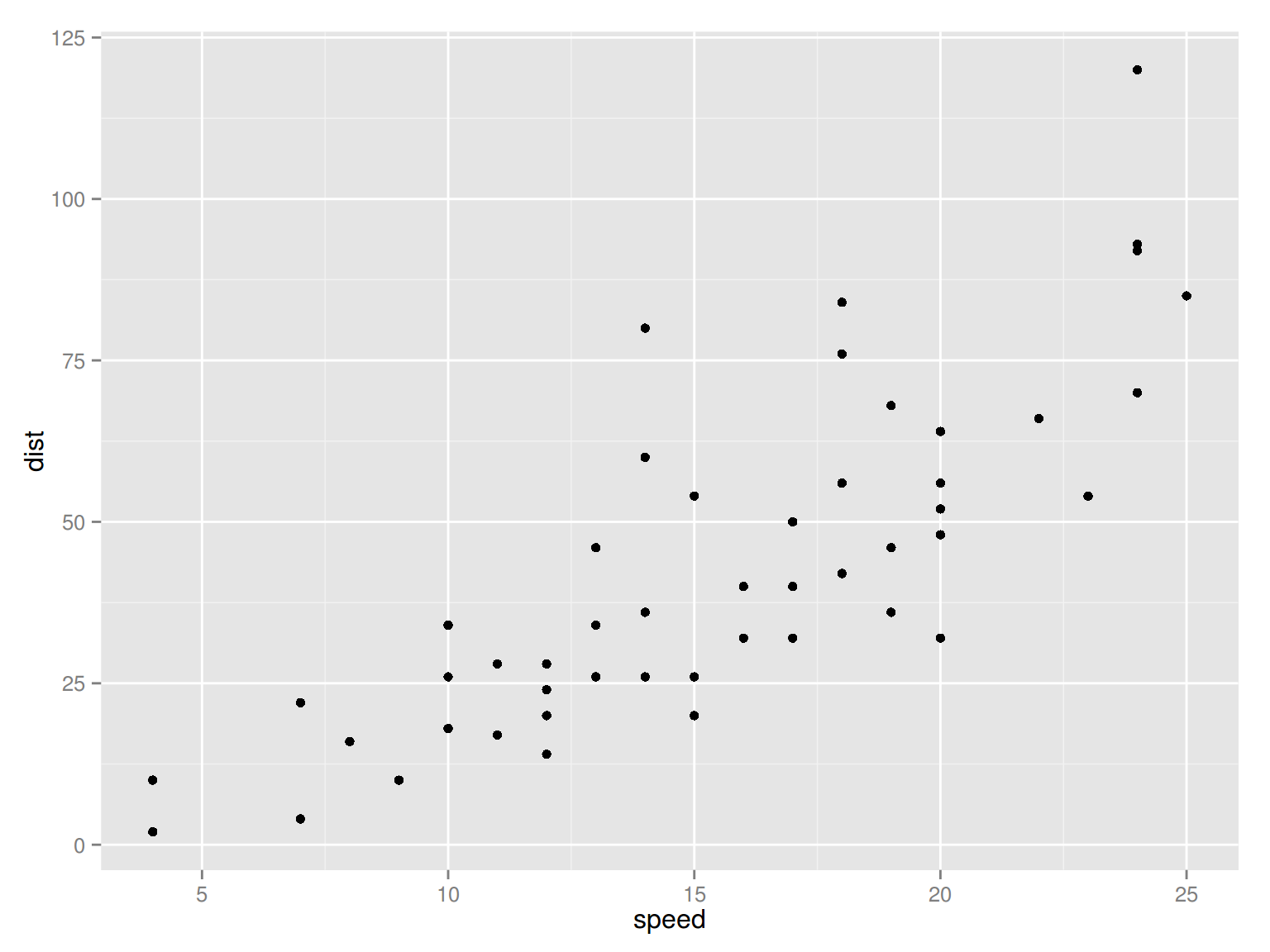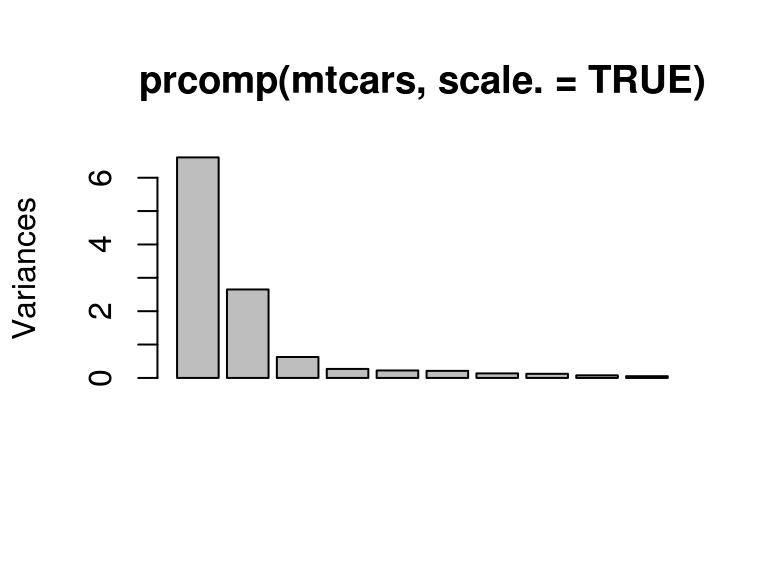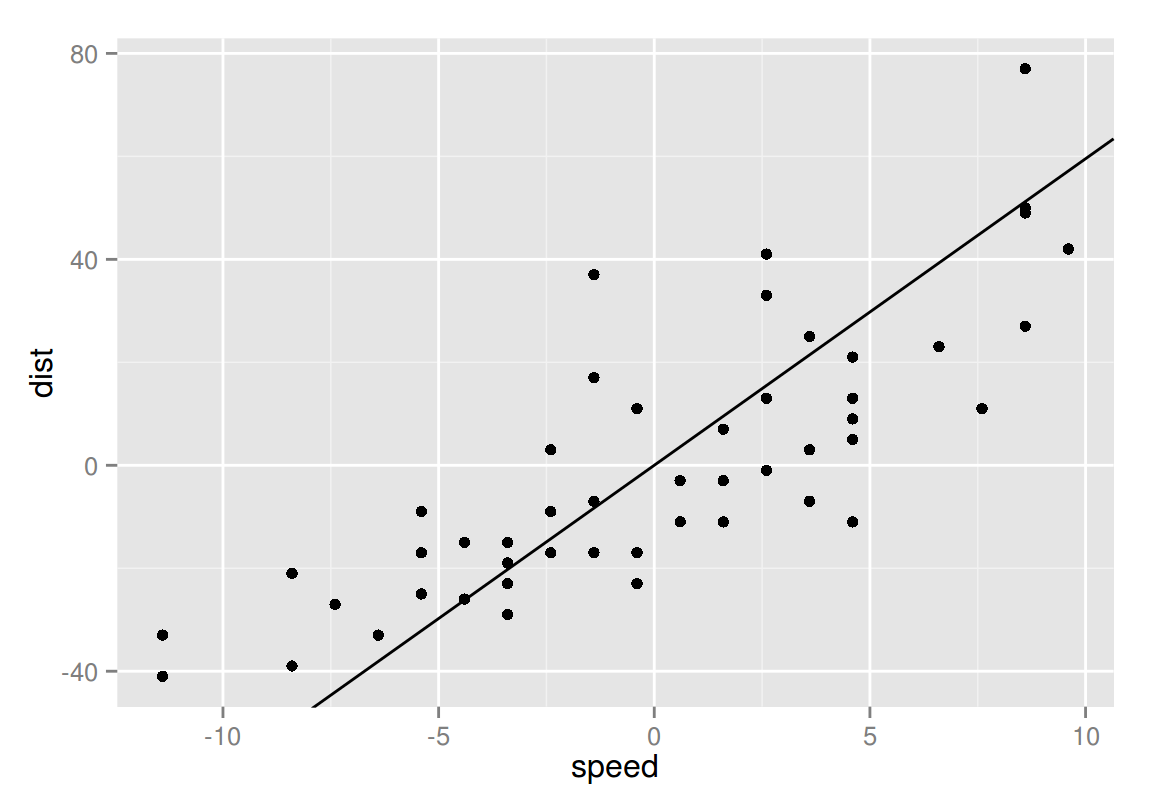March 12, 2015

# PCA and EFA

### Principal Components Analysis (PCA):

• Dimension reduction technique
• Example use case: regression in the presence of multicollinearity
• $$\textbf{PC} = \mathbf{\alpha x}$$
• Iteratively select principal component that accounts for the maximum variance

### Exploratory Factor Analysis (EFA):

• Latent variable identification
• Example use case: indirectly measure concepts such as intelligence that cannot be measured directly
• $$\mathbf{x} = \mathbf{\Lambda f + u}$$
• Select $$\mathbf{\Lambda}$$ to best fit the sample covariance matrix

# Principal Components Analysis (PCA)

• Primary purpose is as a dimension reduction technique
• Goal is to transform a set of correlated variables into a much smaller set of uncorrelated variables
• Each principal component is a linear combination of the input variables:

$\text{PC}_1 = \alpha_{11}x_1 + \alpha_{12}x_2 + \cdot\cdot\cdot + \alpha_{1n}x_n$ $\vdots$ $\text{PC}_k = \alpha_{k1}x_1 + \alpha_{k2}x_2 + \cdot\cdot\cdot + \alpha_{kn}x_n,$

where $$k << n$$

• The principal components are determined iteratively, starting with $$\text{PC}_1$$, then $$\text{PC}_2$$, etc. The coefficients above are chosen to maximize the variance explained while satisfying the requirement that the components be uncorrelated.

# PCA Steps

1. Run prcomp (or princomp)
2. Examine output, summary, and plot of (1)
3. Determine how many components to keep
• Based upon topical knowledge/experience
• Account for some threshold cumulative proportion of variance
• Locate the “elbow” of the scree plot
• Keep all principal components with above average variance (variance > $$1$$ when working with scaled data)

# Hands-on Example: Raw Data

library(ggplot2)
qplot(data=cars, x=speed, y=dist)# Hands-on Example: PCA Output

pca <- prcomp(cars)
print(pca)
## Standard deviations:
##  26.12524  3.08084
##
## Rotation:
##              PC1        PC2
## speed -0.1656479 -0.9861850
## dist  -0.9861850  0.1656479
• “Standard deviations” shows the square roots of the eigenvalues
• “Rotation” shows the eigvenvectors (these provide the $$\alpha$$ coefficients shown earlier in the presentation)
• You can confirm by computing the inner product that the two principal components shown in the “Rotation” output are indeed orthogonal

# Hands-on Example: Summary

print(summary(pca))
## Importance of components:
##                            PC1     PC2
## Standard deviation     26.1252 3.08084
## Proportion of Variance  0.9863 0.01372
## Cumulative Proportion   0.9863 1.00000

Principal component 1 accounts for nearly all of the variance due to the fact that the variances of the two columns are quite different. In general, one should scale their data to have unit variance by passing “scale.=TRUE” to prcomp.

# Hands-on Example: Scree Plot

plot(pca)Normally, you would be considering more than two components and the scree plot would look more like:# Hands-on Example: First Component

ev.slopes <- pca$rotation[2, ]/pca$rotation[1, ]
cars.centered <- transform(cars, speed=speed-mean(speed),
dist=dist-mean(dist))
qplot(data=cars.centered, x=speed, y=dist) +
geom_abline(intercept=0, slope=ev.slopes)# Hands-on Example: Commentary

• Scale input data
• Reduce impact of dramatically different variances amongst input variables
• Pass scale.=TRUE to prcomp or cor=TRUE to princomp
• Rescale principal components
• Rotate components
• Eases interpretation, but components no longer “principal” components
• Orthogonal: varimax, quartimax
• Oblique: oblimin, promax
• See package GPArotation
• Project original data onto components
• Referred to as “scores”
• See princomp$scores or prcomp$x

# Going Further with PCA

Check out the psych package

Provides:

• principal()
• fa.parallel()
• fa()
• …and more!

# Exploratory Factor Analysis (EFA)

• Collection of methods designed to uncover the latent structure in a given set of variables
• Factors are assumed to underlie the observed variables: $x_1 = \lambda_{11} f_1 + \lambda_{12} f_2 + \cdot\cdot\cdot + \lambda_{1n} f_n + u_1$ $\vdots$ $x_k = \lambda_{k1} f_1 + \lambda_{k2} f_2 + \cdot\cdot\cdot + \lambda_{kn} f_n + u_k$

(Recall that in PCA the assumption was that principal components were a linear combination of observed variables)

• Factor loadings and errors aren’t directly observable, but are inferred from the correlations among the variables
• Determine factor loadings as those that most accurately reproduce the sample covariance matrix

# EFA Steps

1. Choose number of factors
2. Run factanal

# Hands-on Example: Raw Data

head(mtcars)
##                    mpg cyl disp  hp drat    wt  qsec vs am gear carb
## Mazda RX4         21.0   6  160 110 3.90 2.620 16.46  0  1    4    4
## Mazda RX4 Wag     21.0   6  160 110 3.90 2.875 17.02  0  1    4    4
## Datsun 710        22.8   4  108  93 3.85 2.320 18.61  1  1    4    1
## Hornet 4 Drive    21.4   6  258 110 3.08 3.215 19.44  1  0    3    1
## Hornet Sportabout 18.7   8  360 175 3.15 3.440 17.02  0  0    3    2
## Valiant           18.1   6  225 105 2.76 3.460 20.22  1  0    3    1

# Hands-on Example: Determining Number of Factors

sapply(1:3, function(f)
factanal(mtcars, factors=f, method="mle")\$PVAL)
##    objective    objective    objective
## 1.496220e-17 4.047510e-04 2.051923e-01

Find the first number of factors where the p-value is not significant. The results above suggest that we should use 3 factors.

# Hands-on Example: Running factanal

factanal(mtcars, factors=3, method="mle")
##
## Call:
## factanal(x = mtcars, factors = 3, method = "mle")
##
## Uniquenesses:
##   mpg   cyl  disp    hp  drat    wt  qsec    vs    am  gear  carb
## 0.135 0.055 0.090 0.127 0.290 0.060 0.051 0.223 0.208 0.125 0.158
##
##      Factor1 Factor2 Factor3
## mpg   0.643  -0.478  -0.473
## cyl  -0.618   0.703   0.261
## disp -0.719   0.537   0.323
## hp   -0.291   0.725   0.513
## drat  0.804  -0.241
## wt   -0.778   0.248   0.524
## qsec -0.177  -0.946  -0.151
## vs    0.295  -0.805  -0.204
## am    0.880
## gear  0.908           0.224
## carb  0.114   0.559   0.719
##
##                Factor1 Factor2 Factor3
## The p-value is 0.205